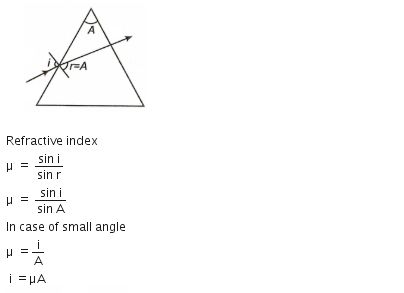# A ray is incident at an angle of incidence i on one surface of a prism of small angle A and emerges normally from the opposite surface. If the refractive index of the material of prism is m, the angle of incidence i is nearly equal to         (a)    A/u         (b)    A/2u         (c)    u A(d)    uA/2

8 years ago

(c)

As refracted ray emerges normally from oppostite surface,

r2 = 0

As A = r1 + r2

.''.     r1 = A

Now, u= sini1/sinr

= i1/r1

=uA

3 years ago
FOR  PRISM ,

A = R1 + R2
As the ray emerges normally,

E = 90 DEGREE  .  R2 = 90
R1  NEEDS TO BE 0 DEGREE

A = R2

U = SIN i/ SIN r

For small angles ,
SIN X  =  X

U  =  SIN i / SIN r
U  =  i / r
U  =  i / A

THUS::::

UA = IYash Chourasiya
one year ago
Dear Student

Please see the solution in the attachment.